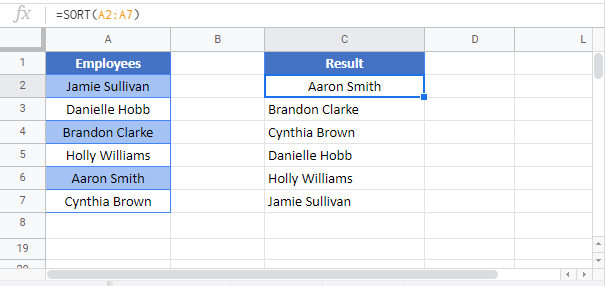# SORT Function Examples – Excel & Google Sheets

This tutorial demonstrates how to use the SORT Function in Excel to sort a list of values.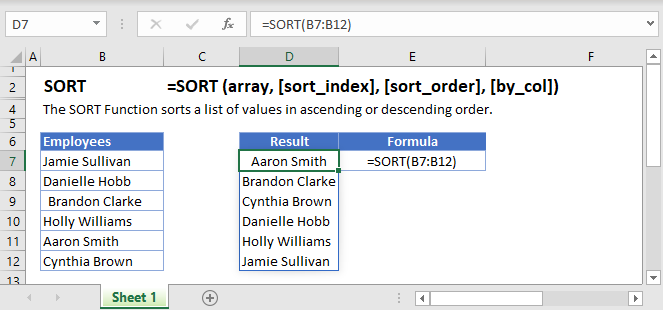## SORT Function Overview

The SORT Function is used to sort values in a range or array. It can be used to arrange values in ascending or descending order.

To use the SORT Excel Worksheet Function, select a cell and type: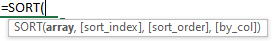(Notice how the formula inputs appear)

## SORT function Syntax and inputs:

array – The range or array to sort.

[sort_index] (optional) – The column index to use for sorting. When omitted it defaults to 1.

[sort_order] (optional)  – Use 1 to sort in  ascending order  and  -1 for descending order. When omitted it defaults to 1 i.e. ascending order.

[by_col]  (optional)  – Use TRUE  to sort by column and FALSE to sort by row. When omitted it defaults to FALSE i.e. Sort by Row.

# How to use the SORT Function

## To sort values in ascending or descending order

We can order an array of values in either ascending or descending order. To do so we manipulate the [sort_order] argument in the SORT Function.

To sort our list of employees in A2:A7 in ascending order we enter the following formula in D2.

`=SORT(A2:A7)`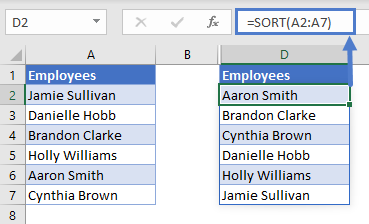You’ll notice that in the example above  we’ve omitted the [sort_order] argument, this is because it isn’t necessary to set its value when you want to order your range in ascending order.

In the example below we’ve set the value of the [sort_order] argument to -1 so as to order our array in descending order. We enter the following formula in D2:

`=SORT(A2:A7, ,-1)`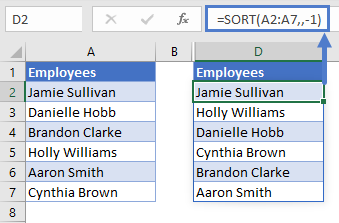You’ll notice that the third argument [sort_order] in our formula  is set to -1. When set to 1 it returns the list in ascending order.

## To sort values by another column

You can also use the SORT Function to sort a column in your array by the values in another column in the same array. For example, we’d like to sort our list of employees in A2:A7 by the departments they work in in B2:B7.  To do so, we enter the following formula in E2:

`=SORT(A2:B7,2)`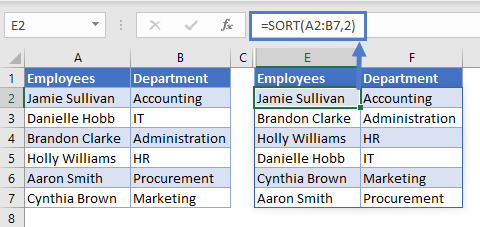You’ll notice that the second argument [sort_index] is set to 2. This means we are using the second column to sort the order of the function’s output.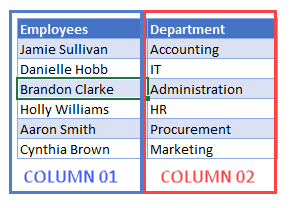This example can also be solved using the SORTBY Function which is closely related to the SORT Function in terms of use case and functionality.

## To sort values by multiple columns

In the previous section we saw how to sort values in one column by values in another column. Using the SORT Function you can go a step further and sort the result by another column.

Imagine a scenario whereby we want to sort our list of employees in A2:A7  by the department they belong to in B2:B7 after which we want to order the names alphabetically within each department. To do so we enter the following formula in E2:

`=SORT(A2:B7,{2,1},{1,1})`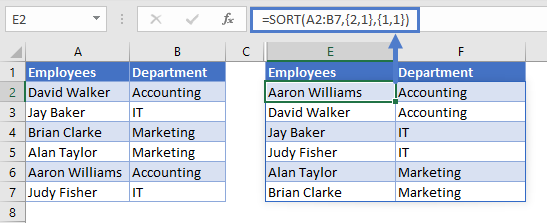We use constant arrays {2,1} and {1,1} to achieve this.

`[sort_index] = {2,1}`

The snippet above means we first sort the data by the second column i.e. the department column and then by the first column i.e. the employee column

`[sort_order] = {1,1}`

The snippet above means we sort both the second and first column in ascending order.

## Use with other Dynamic Array Formulas

The SORT Function can be used along with other Dynamic Array Formulas such as UNIQUE and FILTER for added functionality.

## With UNIQUE Function

For example, we’d like to sort the list of unique employees in our company, to do so we enter the following formula in D2:

`=SORT(UNIQUE(A2:A7))`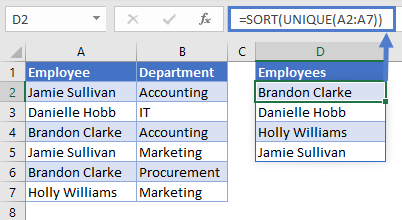## With FILTER Function

For example, we’d like to return a list of fruits whose sale-quantity has hit or exceeded our target in D2,  to do so we enter the following formula in F2:

`=SORT(FILTER(A2:B17,B2:B17>=D2),2,-1)`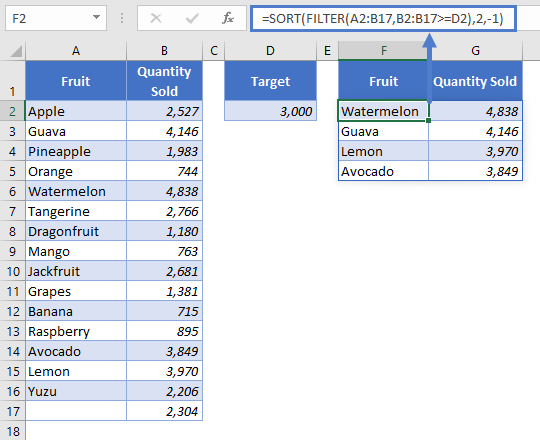## Issues

### #SPILL!

This error occurs when there is a value in the Spill Range i.e. the range where the SORT Function places its results.

To correct this error, clear the range that Excel highlights.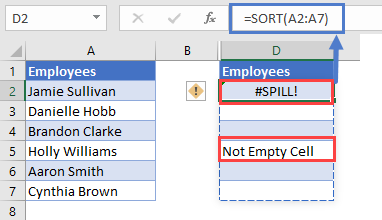### SORT Function Tips and Tricks

1. The [sort_order] can only either be 1(ascending) or -1(descending), when no value is given for this argument the SORT Function defaults to sorting in ascending order.

1. Ensure that the cells below the input cell are blank to avoid the Spill Error, learn more about the Spill Error ‘here’ — add link to “Introduction To Dynamic Array Formulas”1. The SORT Function can be used with other Dynamic Array Functions such as FILTER  and UNIQUE to create more versatile formulas.